VHDL coding tips and tricks: VHDL code for BCD to 7-segment display converter

## Saturday, March 6, 2010

### VHDL code for BCD to 7-segment display converter

Here is a program for BCD to 7-segment display decoder. The module takes 4 bit BCD as input and outputs 7 bit decoded output for driving the display unit.A seven segment display can be used to display decimal digits.They have LED or LCD elements which becomes active when the input is zero.The figure shows how different digits are displayed:

library IEEE;
use IEEE.STD_LOGIC_1164.ALL;
use IEEE.STD_LOGIC_ARITH.ALL;
use IEEE.STD_LOGIC_UNSIGNED.ALL;

entity test is
port (
clk : in std_logic;
bcd : in std_logic_vector(3 downto 0);  --BCD input
segment7 : out std_logic_vector(6 downto 0)  -- 7 bit decoded output.
);
end test;
--'a' corresponds to MSB of segment7 and g corresponds to LSB of segment7.
architecture Behavioral of test is

begin
process (clk,bcd)
BEGIN
if (clk'event and clk='1') then
case  bcd is
when "0000"=> segment7 <="0000001";  -- '0'
when "0001"=> segment7 <="1001111";  -- '1'
when "0010"=> segment7 <="0010010";  -- '2'
when "0011"=> segment7 <="0000110";  -- '3'
when "0100"=> segment7 <="1001100";  -- '4'
when "0101"=> segment7 <="0100100";  -- '5'
when "0110"=> segment7 <="0100000";  -- '6'
when "0111"=> segment7 <="0001111";  -- '7'
when "1000"=> segment7 <="0000000";  -- '8'
when "1001"=> segment7 <="0000100";  -- '9'
--nothing is displayed when a number more than 9 is given as input.
when others=> segment7 <="1111111";
end case;
end if;

end process;

end Behavioral;

If you want a decimal number to be displayed using  this code then convert the corresponding code into BCD and then instantiate this module for each digit of the BCD code.

Here is a sample test bench code for this module:

LIBRARY ieee;
USE ieee.std_logic_1164.ALL;
use IEEE.STD_LOGIC_ARITH.ALL;

ENTITY test_tb IS
END test_tb;

ARCHITECTURE behavior OF test_tb IS
signal clk : std_logic := '0';
signal bcd : std_logic_vector(3 downto 0) := (others => '0');
signal segment7 : std_logic_vector(6 downto 0);
constant clk_period : time := 1 ns;
BEGIN
uut: entity work.test PORT MAP (clk,bcd,segment7);
clk_process :process
begin
clk <= '0';
wait for clk_period/2;
clk <= '1';
wait for clk_period/2;
end process;
stim_proc: process
begin
for i in 0 to 9 loop
bcd <= conv_std_logic_vector(i,4);
wait for 2 ns;
end loop;
end process;

END;

#### 30 comments:

1.hello.. can you pls post a test bench for this code.. tnx...

2.@Alfred : I have modified the post including the test bench.Hope that helps..

3.tnx a lot..

4.thank you, it is so useful for me.
Can you show me, how to control a animation on vga using keyboard in vhdl

5.Hi, I'm trying to implement the sum of two numbers with 5 bits and show in two digits SSD, but with no success, do you have any idea how to do this?

I had success with decimal counter until 99, but how make this work whit the sum, I don't know.

Thanks

6.Can some one help me with the code for Four bit BCD decimal COUNTER using VHDL and the 74LS90. I'm using Xilinx 12.1 and I'm really struggling with the logic gate code.
my email is jct0378@gmail.com

7.Please help me!

Write a VHDL code to perform the function of multiplier
which the inputs are from Dip Switch and outputs
display to 7-segment LED with BCD.
X : dip 1~4represents value 0~15
Y : dip 5~8represents value 0~15

Thanks you so much

8.write a VHDL prog to display number on BCD-7 segment display , input given from ps/2 keyboard

9.can you help me to get a VHBL program for 64 bit CSA

10.Can you post the synthesis report.

11.Hi, need help can you please provide me the VHDL codes for "SN74LS247" which is BCD 2 7-segment decoder/driver similar to one mention above but it has 3 additional logic and no clock.
plz help me or u can send me code on desai335@yahoo.com.
This will be really appreciated.

12.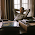can you post code for multiplexed display

13.can anyone send me the project which consists ASIC design that are using synthesis tool and VHDL code. I'm new from this field can anyone send overall view to design the project. what the companies can do regarding ASIC design (overall) send to email address arun.distinctive@gmail.com

please send it asap............and overall project

14.In reply to @Bhaumik:

library IEEE;
use IEEE.STD_LOGIC_1164.ALL;

entity SN74LS247 is
Port ( BCD_I : in STD_LOGIC_VECTOR (3 downto 0);
RBI : in STD_LOGIC;
LT : in STD_LOGIC;
BI : in STD_LOGIC;
RBO : out STD_LOGIC;
SEG_O : out STD_LOGIC_VECTOR (6 downto 0));
end SN74LS247;

architecture Behavioral of SN74LS247 is
signal enable : std_logic;
begin

enable <= LT and BI;

--abcdefg
SEG_O <= "1111110" when (BCD_I = "0000") and enable = '1' and RBI = '1' else -- 0
"0110000" when (BCD_I = "0001") and enable = '1' else -- 1
"1101101" when (BCD_I = "0010") and enable = '1' else -- 2
"1111001" when (BCD_I = "0011") and enable = '1' else -- 3
"0110011" when (BCD_I = "0100") and enable = '1' else -- 4
"1011011" when (BCD_I = "0101") and enable = '1' else -- 5
"1011111" when (BCD_I = "0110") and enable = '1' else -- 6
"1110000" when (BCD_I = "0111") and enable = '1' else -- 7
"1111111" when (BCD_I = "1000") and enable = '1' else -- 8
"1111011" when (BCD_I = "1001") and enable = '1' else -- 9
"0001101" when (BCD_I = "1010") and enable = '1' else -- 10
"0011001" when (BCD_I = "1011") and enable = '1' else -- 11
"0100011" when (BCD_I = "1100") and enable = '1' else -- 12
"1001011" when (BCD_I = "1101") and enable = '1' else -- 13
"0001111" when (BCD_I = "1110") and enable = '1' else -- 14
"0000000" when (BCD_I = "1111") and enable = '1' else -- 15
"0000000" when RBI = '0' and LT = '1' and BCD_I = "0000" and BI = '0' else
"0000000" when BI = '0' else
"1111111" when LT = '0' else
"0000000";

RBO <= '1' when LT='1' and RBI='0' and BCD_I="0000" else '0';

end Behavioral;

1.Urgent...
I need test bench for this please,

library IEEE;
use IEEE.STD_LOGIC_1164.ALL;

entity SN74LS247 is
Port ( BCD_I : in STD_LOGIC_VECTOR (3 downto 0);
RBI : in STD_LOGIC;
LT : in STD_LOGIC;
BI : in STD_LOGIC;
RBO : out STD_LOGIC;
SEG_O : out STD_LOGIC_VECTOR (6 downto 0));
end SN74LS247;

architecture Behavioral of SN74LS247 is
signal enable : std_logic;
begin

enable <= LT and BI;

--abcdefg
SEG_O <= "1111110" when (BCD_I = "0000") and enable = '1' and RBI = '1' else -- 0
"0110000" when (BCD_I = "0001") and enable = '1' else -- 1
"1101101" when (BCD_I = "0010") and enable = '1' else -- 2
"1111001" when (BCD_I = "0011") and enable = '1' else -- 3
"0110011" when (BCD_I = "0100") and enable = '1' else -- 4
"1011011" when (BCD_I = "0101") and enable = '1' else -- 5
"1011111" when (BCD_I = "0110") and enable = '1' else -- 6
"1110000" when (BCD_I = "0111") and enable = '1' else -- 7
"1111111" when (BCD_I = "1000") and enable = '1' else -- 8
"1111011" when (BCD_I = "1001") and enable = '1' else -- 9
"0001101" when (BCD_I = "1010") and enable = '1' else -- 10
"0011001" when (BCD_I = "1011") and enable = '1' else -- 11
"0100011" when (BCD_I = "1100") and enable = '1' else -- 12
"1001011" when (BCD_I = "1101") and enable = '1' else -- 13
"0001111" when (BCD_I = "1110") and enable = '1' else -- 14
"0000000" when (BCD_I = "1111") and enable = '1' else -- 15
"0000000" when RBI = '0' and LT = '1' and BCD_I = "0000" and BI = '0' else
"0000000" when BI = '0' else
"1111111" when LT = '0' else
"0000000";

RBO <= '1' when LT='1' and RBI='0' and BCD_I="0000" else '0';

end Behavioral;

my email is :
yns69167@gmail.com
15S13544@mec.edu.om

15.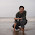how to i show the otuput of this above program using quartus II

16.please help me i need vhdl code for mimo using communication system

17.i need vhdl coding for mimo using communication system

18.hi could you please create a TEST BECH for SN74LS247...

1.kindly share the test bech code

19.hi , is the clock necessary for this code ?

20.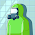hi , is the clock necessary for this code ?

21.hie can anyone help me to blink an external led using spartan 3e using vhdl

22.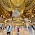help me, i need vhdl code for counter for atm machine when login more than 3 times its terminate . please urgent :(

23.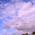Hi ,,do you know how to do vhdl code for 2 bit x 2 bit multiplier using decoder to seven segment display?? Please please help me..i seriously dont know how to do it.

24.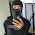hi i need to code 7segment BCD by use if , else. Please help me and thank for answer.

25.how do you display on the 7-segment display and display using the led's to display the binary value at the same time

26.do you have the corresponding XDC file ?

27.If block diagram of binary to 7segment is given , much easier to understand.
Plz. If anyone can post the block diagram and vhdl code in behavioural modelling for this .

28.Can you share UCF File ?